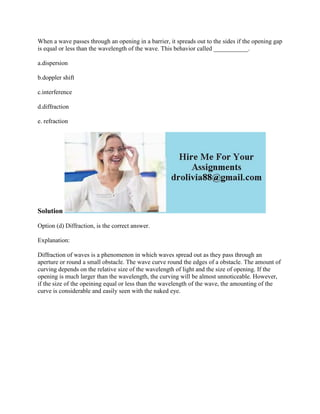Diese Präsentation wurde erfolgreich gemeldet.

# When a wave passes through an opening in a barrier- it spreads out to.docx

Anzeige
Anzeige
Anzeige
Anzeige
Anzeige
Anzeige
Anzeige
Anzeige
Anzeige
Anzeige
Anzeige×

1 von 2 Anzeige

# When a wave passes through an opening in a barrier- it spreads out to.docx

When a wave passes through an opening in a barrier, it spreads out to the sides if the opening gap is equal or less than the wavelength of the wave. This behavior called ___________.
a.dispersion
b.doppler shift
c.interference
d.diffraction
e. refraction
Solution
Option (d) Diffraction, is the correct answer.
Explanation:
Diffraction of waves is a phenomenon in which waves spread out as they pass through an aperture or round a small obstacle. The wave curve round the edges of a obstacle. The amount of curving depends on the relative size of the wavelength of light and the size of opening. If the opening is much larger than the wavelength, the curving will be almost unnoticeable. However, if the size of the opeining equal or less than the wavelength of the wave, the amounting of the curve is considerable and easily seen with the naked eye.
.

When a wave passes through an opening in a barrier, it spreads out to the sides if the opening gap is equal or less than the wavelength of the wave. This behavior called ___________.
a.dispersion
b.doppler shift
c.interference
d.diffraction
e. refraction
Solution
Option (d) Diffraction, is the correct answer.
Explanation:
Diffraction of waves is a phenomenon in which waves spread out as they pass through an aperture or round a small obstacle. The wave curve round the edges of a obstacle. The amount of curving depends on the relative size of the wavelength of light and the size of opening. If the opening is much larger than the wavelength, the curving will be almost unnoticeable. However, if the size of the opeining equal or less than the wavelength of the wave, the amounting of the curve is considerable and easily seen with the naked eye.
.

Anzeige
Anzeige

### When a wave passes through an opening in a barrier- it spreads out to.docx

1. 1. When a wave passes through an opening in a barrier, it spreads out to the sides if the opening gap is equal or less than the wavelength of the wave. This behavior called ___________. a.dispersion b.doppler shift c.interference d.diffraction e. refraction Solution Option (d) Diffraction, is the correct answer. Explanation: Diffraction of waves is a phenomenon in which waves spread out as they pass through an aperture or round a small obstacle. The wave curve round the edges of a obstacle. The amount of curving depends on the relative size of the wavelength of light and the size of opening. If the opening is much larger than the wavelength, the curving will be almost unnoticeable. However, if the size of the opeining equal or less than the wavelength of the wave, the amounting of the curve is considerable and easily seen with the naked eye.